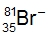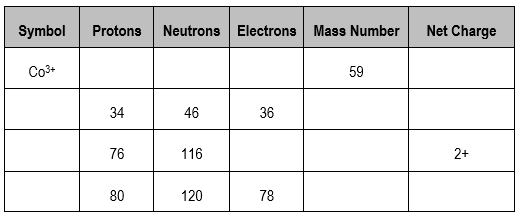## General Chemistry

Learn the toughest concepts covered in Chemistry with step-by-step video tutorials and practice problems by world-class tutors

2. Atoms & Elements

# Ions

Ions represent elements that possess either a positive charge or a negative charge.

Determining Ionic Charge
1
concept

## Ions Concept 11m
Play a video:
At this point, we have just examined Adams, where we're dealing with the neutral element that has the same number of protons and electrons. Now, when the number of protons and electrons are different, that's what we go into ions. Ions themselves are created from loss or gaining of electrons by elements. So in the creation of an ion, or either losing electrons or gaining electrons, we're going to stay here. When an element loses electrons, it becomes a positively charged ion called a cat ion. Now this makes sense. You're losing negatively charged electrons. If I'm losing something negative, I'm becoming more positive now. On the other end, when an element gains electrons so it's gaining negative electrons, it becomes a negatively charged ion called in an ion. So just remember, cat ions are positively charged. Ions and ions are negatively charged ions. We're going to stay here a new term. Isil electronic. This is what elements have the same number off electrons. So just remember the difference between atoms and ions is that atoms have the same number of protons and electrons. But when we become an ion, we've either lost electrons or gained electrons. This causes a difference in the number of protons and electrons within a given element, so keep that in mind when dealing with ions.

Ions are formed from either the loss or gaining of electrons by a neutral element.

2
concept

## Ions Concept 26m
Play a video:

A positively charged ion is called a cation and a negatively charged ion is called an anion

3
example

## Ions Example 12m
Play a video:
So here in this example question, it states determine the number of protons, neutrons and electrons for the following cat ion. Now it's a cat ion because it possesses a positive charge, that positive charge being three plus now, when looking at the other numbers, we know that this 13 here represents our atomic number, which uses the variables. E remember the atomic number gives us the number of protons, and because it's 13, that means we have 13 protons. That means that option. He cannot be an answer, because here we do not have 27 protons. Next, we have the number above the 13 and 27. Remember, that is your mass number, which uses the variable A. Your mass number gives you the number of protons plus neutrons within the element or ion. From this information, we know that the number off neutrons would equal a minus Z, so it's the mass number minus the atomic number, So that would be 27 minus 13, which would give us 14 neutrons. So so far, options B and C can be the only correct choices because in a we do not have 27 new trials. Now the charge is three plus three plus means that you have lost electrons. It means you have lost three electrons. When aluminum is neutral has no charge. It has an equal number of protons and electrons. Okay, but now we've lost three electrons. So what does that mean? That means we have 10 electrons remaining. So the answer would have to be see here. And if you want to double check that, you could just say we have 13 protons and 10 electrons, so we know that would be plus 13 plus minus 10, which would give us plus three as an answer. That proves that our charge should be plus three here. So, out of all the choices present Onley option C gives the correct number for each of the subatomic particles.
4
Problem

Give the correct number of protons, neutrons and electrons for the following isotope:.

5
Problem

In which pair are the two species both isoelectronic and isotopic?

6
Problem

One isotope of a metallic element has a mass number of 65 and 35 neutrons in the nucleus. The cation that this atom forms has 28 electrons. What is the symbol of the cation?

7
Problem

Which of the following is the symbol for the ion with a +4 charge, 30 neutrons and 21 electrons?

8
Problem

Fill in the gaps for the following table.